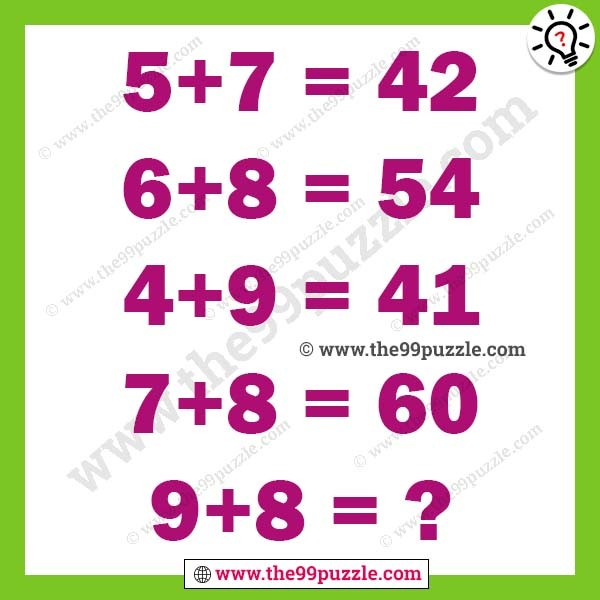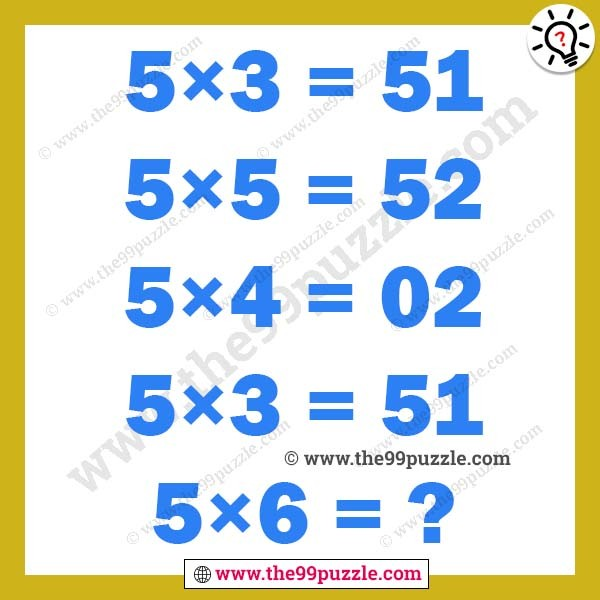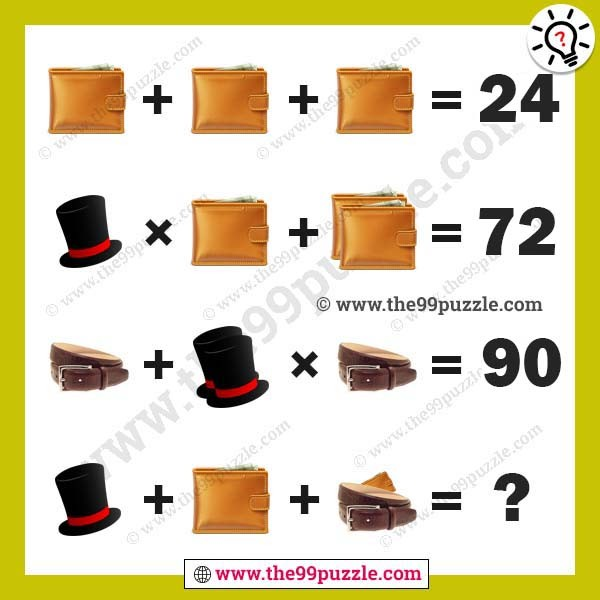# 9 Logic Puzzles that will test your brain power

9 Logic Puzzles that will test your brain power. Here you will get many brain teasers logic puzzles. All these reasoning puzzles are very challenging to solve. If you are a puzzles lover you can easily solve these. Nowadays logical puzzles are asked in many examinations and interviews. If you are a genius you can answer all of these logic puzzles.

1. Logical Math Equation with Answer for Teens

5+7=42, 6+8=54, 4+9=41, 7+8=60, 9+8=?2. What number can replaces the question mark?3. Logical reasoning math puzzles for adults

5×3=51, 5×5=52, 5×4=02, 5×3=51, 5×6=?4. Which letter should replace the question mark?5. Math picture equation for genius with answer6. Fun and Challenging Math Riddles With Answers7. Wallet, cap, belt picture puzzles equation for genius8. Mathematics equations picture puzzles for genius with answers9. Brain teaser letter puzzles for genius

3+8+2=M, 5+1+9=O, 7+4+3=N, 9+3+4=?Here you will find many different types of math puzzles logic for adults and kids. 9 Logic Puzzles that will test your brain power. Get more interesting tricky puzzles, riddles, brain teasers, brain games, picture puzzles, maths puzzles, and many more.